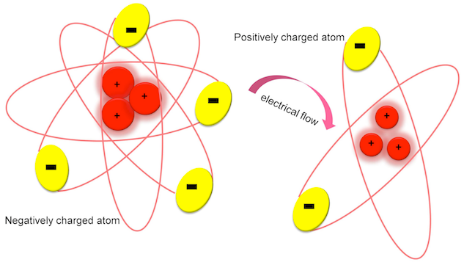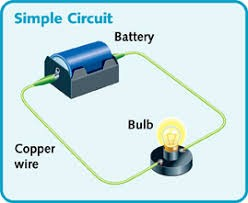Click to Chat

1800-1023-196

+91-120-4616500

CART 0

• 0

MY CART (5)

Use Coupon: CART20 and get 20% off on all online Study Material

ITEM
DETAILS
MRP
DISCOUNT
FINAL PRICE
Total Price: Rs.

There are no items in this cart.
Continue Shopping• Complete Physics Course - Class 11
• OFFERED PRICE: Rs. 2,968
• View Details

```Int  Introduction of Current Electricity

Table of Content

Introduction

History

Static Electricity

Current Electricity

Circuit

Parameters in a circuit

Ohm’s Law

Summary

Introduction

We know that atoms are made up of protons, electrons and neutrons. Protons and Neutrons are placed at the center of the atom which is called the Nucleus. Electrons moves around the nucleus. These electrons possess some energy. Neutrons does not have any charge and protons are positively charged. Electrons are negatively charged. Electrons circles the nucleus as opposite charges (protons and electrons) attract each other. The electrons moves from one atom to another. Thus electricity is produced when protons and electrons interact each other. It is mainly the flow of electrons. Electricity is of two forms –  Static Electricity and Current Electricity.

Atoms in a conductor consists of free electrons that moves gently between the atoms. The movement of these electrons in the atoms are irregular and undirected. This means that there is no flow in any particular direction. When we apply voltage to the conductor these free electrons moves in the same direction and thus creates current. So current is the flow of electrons, the charged particles through a conducting medium.Flow of electrons

History

Thales of Miletus introduced the concept of electric power in nature. He was a Greek philosopher who discovered the notion of static electricity by rubbing amber with a section of fur. Amber is a type of fossilized wood. This amber when rubbed with fur or cloth will attract small pieces of dust and others. This ability to attract other objects is caused by the effect of static electricity. The word electricity is arrived from ‘elektron’ which means amber. It was introduced by William Gilbert. He also discovered the science of magnetism. He claimed that electricity and magnetism are entirely different and this led to the introduction of electromagnetism.

Later Benjamin Franklin stated that electric charge is of two forms-positive and negative. He found out that lightning was electrical by doing the kite experiment. He attached the kite to a silk string and tied a key to the other side. He then inserted a wire to a Leyden jar to collect the electric charge. During thunderstorm Franklin flew the kite and as the thunderstorm passed through the kite, the negative charges were collected and it filled the jar. He was insulated by holding the dry silk ribbon. The experiment proved that lightning is static electricity.Kite experiment

Electric Current was not fully looked into up to the time batteries were designed. Alessandro Volta invented electric battery. It was first named as voltaic pile. For his contributions to science, the unit of electric potential is named as Volt. Michael faraday, another great scientist has made major discoveries in the field of electricity. One of his finest discovery is electromagnetic induction. He found out that varying magnetic fields produce electricity in an electric circuit. He mentioned that kinetic energy can be converted into electrical energy using electromagnetic induction. This is the principle behind electric transformer and generator. He also developed electric motor.

Thomas Alva Edison who invented electric bulb, was also a great scientist and this invention was an important milestone in the field of electricity. The direct current (dc) system of generating power was invented by Edison. Nikola Tesla invented the alternating current (ac) system and thus he developed ac motor. Now AC current system is commonly used everywhere.

Static and Current Electricity

There are two types of electricity, Static Electricity and Current Electricity.

Static Electricity

Consider two objects and when these two objects are rubbed together, one material gives up electrons and the other one collects those electrons. The one which leaves the electrons becomes more positively charged and the other one which receives the electrons becomes more negatively charged. This accumulation of more charge is called Static Electricity. Static Electricity has high voltage and low current.

Lightning is another example for static electricity. It happens due to the attractions of opposite charges which is formed by the friction between air, water droplets and ice particles. Static electricity is used in Xerox machines, laser printers, crystal microphones etc. However, the discharge of static electricity may cause severe dangers in industries with flammable substances. Electric shock may appear while touching an object with high electric charge.Lightning formation

Current Electricity

Electrical Current is the flow of charged particles. The flow of charges will be constant in current electricity. For the current to flow we need a circuit. The electrons in a current flows from negative to positive. Electrons flows in the direction which is opposite to the direction of electric current. Electric current flows from higher electric potential to lower electric potential. Electric Current is divided into two - Direct Current (DC) and Alternating Current (AC). In Direct Current, the electric charge flows in one direction. In Alternating Current, the direction of the electric charge changes sporadically. DC current is obtained from cell or battery. AC current is obtained from AC generator and mains.

For the current to flow, it requires a circuit which is closed loop of a conducting material. It consists of wires which are connected end to end and the electrons flow in same direction.

Circuit

A circuit has conductors (wire), switch, load and a power source. A circuit starts and stops at the same point. Usually copper wires are used as conductors without insulation. It is through the conductor the current flows. A switch is used to open or close the circuit. When the switch is closed current flows through the circuit and when the switch is open, it breaks the circuit and no current flows through it. The load which is also known as Resistor uses the electrical energy and converts it into some other form of energy. It can be a light bulb or anything else. If there is no load in the circuit then it will be a short circuit. A cell can be the power source. If we put more than one cell it becomes a battery.

There are mainly two types of circuits, series and parallel. In series circuit electrons travel only in one path. In parallel circuit electrons travel through many branches in it. Both series and parallel circuits consists of more than one load.Parameters in a circuit

Electric Current is measured in amperes (amps) by an ammeter which will be connected in series with other components in a circuit. Current, I = Q / t, where Q is the charge in coulombs and t is the time in seconds. Andre Marie Ampere found that two parallel wires attract each other when the electric current flows in one direction. Also two parallel wires repels one another when the electric current flows in opposite directions. As a result of his discoveries in this area, the unit of current is derived from his name which is called as ‘amp’. One amp is equal to one coulomb of charge per second of time.

Voltage is defined as the electrical potential difference between two points in a circuit. The unit of voltage is volts. The unit is derived from the name Alessandro Volta. Cells or batteries provide the voltage or the potential difference which is required.

Resistance resists the flow of current. It is the measure of an objects ability to hold off the flow of electrons. The resistance will be low in a conductor and high in an insulator. It is measured in ohms. The unit ohm is named after the scientist Georg Simon ohm who formulated the ohm’s law. Resistors are used to control the flow of electric current in a circuit. It converts electrical energy into heat and light.

Ohm’s Law

Georg Simon ohm showed the relationship between voltage, current and resistance and formulated the ohm’s law. This law is the basis of electricity.

The law states that V = I R, where voltage V is in volts, Current I in amps and resistance R in ohms.

Thus I = V / R and R = V / I.

For more clarity, electricity can be related to water. Thus the voltage in a circuit whose unit is volts is identical to the pressure of water which is flowing in a pipe. The current in a circuit, where the unit is amps, is equivalent to the water flowing through a pipe. The resistance in the circuit, whose unit is ohms is same as that of the friction and size of the pipe which restricts the flow the water.

Summary

Electricity is produced due to the flow of electrons within an atom of a matter.

Electricity is divided into two-Static electricity and Current electricity.

The word electricity is derived from ‘elektron’ which means amber.

William Gilbert introduced the concept of electromagnetism.

Benjamin Franklin conducted the kite experiment and discovered that lightning was electrical which is static.

Michael Faraday’s main discovery is electromagnetic induction.

Electric bulb which is invented by Thomas Alva Edison has provided many contribution in the field of electricity.

Nikola Tesla invented the alternating current (ac) system and Thomas Edison invented the Direct Current (dc) system.

Static electricity is the collection of more charge when objects are rubbed together.

Electrical current is the flow of charged particles in a circuit.

A circuit consists of a conductor, load, switch and power source.

Current, I = Q / t, where Q is the charge in coulombs and t is the time in seconds.

Voltage is the electrical potential difference between two points in a circuit. Volt is the unit of voltage.

Resistance is the ability to control the flow of electrons in a circuit and the unit of resistance is ohms.

Ohm’s law states that V = I R. Thus I =V / R and R = V / I.

Introduction of Current Electricit
```### Course Features

• 101 Video Lectures
• Revision Notes
• Previous Year Papers
• Mind Map
• Study Planner
• NCERT Solutions
• Discussion Forum
• Test paper with Video Solution